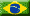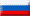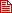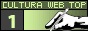agoniaenglishv3 Agonia.Net | Policy | Mission Contact | ParticipateArticle Communities Contest Essay Multimedia Personals Poetry Press Prose _QUOTE Screenplay SpecialTexts by the same author

Translations of this text
0

The influences of Egyptian mathematics on Pythagorean arithomosophy
essay [ ]
about the relationship between mathematics and philosophy

- - - - - - - - - - - - - - - - - - - - - - - - -
by [Sydonay ]

2012-04-10  |     |

I make these specifications because of some incompleteness, but especially because of an error that I encountered reading Kabbalistic interpretation of the meanings of numbers.

Pythagorean philosophy was the theoretical product of one of the most prolific schools of thought of humanity. It is quite possible that the philosophical conceptions developed by this school of thinking have been affected by Oriental conceptions from Antique China and Indian subcontinent.

‘Italian philosophers’, how were termed members of this philosophical branch belonging to ancient Greek cultural space, had found meanings for each number, as for each class or category of numbers which were considered more or less important.

Thus, they asserted that ‘one’ and ‘two’ weren’t numbers in the proper sense of the word, but they played two essential distinct functions. They were generating principles of numbers, this from a mathematical point of view, and, as well, they were constituents of reality, this from an ontological perspective.

‘One’ was also named ‘Monas’, word which in ancient Greek means, as noun, ‘Unity’, and, as adjective, ‘unique’, while the concept of ‘Two’ was termed ‘Dyas’, which means Duality or Dyad.

From these mathematical generating principles, Monas and Dyas, it was inferred the whole series of natural numbers. The odd numbers were considered as being masculine, and even numbers, feminine. From a Pythagorean point of view, the masculine principle has some features as detachment, perfection and finiteness, while the feminine principle has its own opposite properties, as participation (methesis), imperfection and infinity. That means that these philosophers identified finite with perfection and, converse, infinity with imperfection, which seems to be an obvious pre-Christian doctrine. Similar connections with those of Pythagorean philosophers we may find in Chinese philosophy, where the masculine principle, yang, is considered qualitative superior to the feminine principle, yin, thanks to its creative and active attributes, but, even so, it is still necessary to be done the significant difference between Chinese philosophy and Pythagorean school, which was quite unique in the history of thinking because only the pupils of Pythagora stated that finiteness it is (a) perfection.

Therefore, if we would talk about an import of ideas which Pythagorean school would had been made from an Eastern philosophy or metaphysics we could, more probably, to think at Vedic orthodox philosophies as Samkhya-Yoga, and, especially, at certain traditions as shaivism or Shakta school because just there still exist conceptions where masculine principle it is detached from material realm (though, neither in those doctrines finiteness isn’t associated with perfection). For example, in shaivism, Shiva is the embodiment of the male creative power, while the feminine principle, Kali, it is identified with the world, taking part forever to the perpetual illusion which world proves to be.

One or Monas is, in this way, the main numerical principle and, in the same time, masculine principle, while Two or Dyad it is the second mathematical principle after One and the feminine principle, as well. Thinking in this manner, three will be considered as being the first number, in accordance with the most of the commentators.

Therefore, in terms of methodology, each number it is produced from Monas, which, through its self-referential application will generate and, in the same time, will justify the Dyad. After this retrospective action- knowing that the mathematical principles are prior to any human computation or concrete existence- the Dyad will apply to itself producing the number two, becoming determined in this way. After that, to infer superior numbers, we will reconstitute the number two and from it we will achieve number four, after another application of the same Dyad to the number two. This progression could be continued indefinitely, in this way being produced numbers eight, sixteen, and so on. Monas, otherwise implicated just in the process of generating indirectly the number two, will be involved in an explicit way in producing all odd numbers through its addition to each even number. Of course, not every even natural number could be inferred from the final addition of Dyad, at least not those even numbers which aren’t produced through the successive doubling of two and of its derivatives. In this case, for producing numbers six or ten, for example, will be necessary, in the first place, to generate the number three and to apply the Dyad again to this number

The Pythagorean method presents some methodological oddities and hermeneutical issues because of its claim to metaphysically justify the numbers and, as I will show to you in the last part of my article, because of its obvious substantiation from Egyptian mathematics, at least from my point of view.

So, the way in which arithmetical theory of Pythagoreans it is shaped affects the process of generating natural numbers, each member of the series being derived as all the other numbers had not existed prior to the time of the process of generating a given number.

Every principle from Pythagorean philosophy of numbers, named also arithmosophy, had certain conceptual implications. Therefore, One it is, in addition to aforementioned attributions, the formal principle of the point, knowing that the point it is dimensionless, just as One it is an extra-mathematical principle of the numbers. But, as well, from an ontological approach, One it is the supreme cosmic force, natura naturans.

The Dyad it is the formal principle of the line, geometrical construction with a single dimension, but, besides that, the Dyad it is the natural and mathematical principle which intermediates the connection between Oneness and plurality because just with the intervention of the Dyad becomes possible any movement, any cosmological process, knowing that the first spatial dimension is the base for building the whole physical world.

The Triad it is notional expression of the first odd number, three, and, as well, the formal principle of bi-dimensional plane. That means that this numerical concept designates geometrical corporality, not the natural one.

The Tetrad denotes number four and it is the formal principle of the solid bodies, of the tri-dimensional bodies from nature.

As I stated above, Pythagorean arithmosophy it was determined, almost for sure, by Antic Egyptian mathematics, more exactly, by the method of multiplication used in ancient Egypt of pharaons, this method itself deriving from an esoteric philosophy of numbers.

For ancient Egyptians the formal equivalents of the multiplication table were the following mathematical operations. Thus, for the first term of the multiplication, the equivalent in the Egyptian system of computing were the numbers generated through successive doublings beginning from one, while the second term of the multiplication corresponded to numbers produced doubling the second term itself, as you can see in the example from below:

12X11=132

1 11

2 22

4 44

8 88

Seeing that 8+4=12, which corresponds to the first term of multiplication, and 44 and 88, in their column, corresponds, if we would draw a horizontal line to unite the same level terms from these two columns, to 4, respectively, to 8. Also, the amount of 44 and 88 will be 132, which is exactly the result of this multiplication.

As you can see, the factor which makes possible that the numbers from the left column to produce both odd and even numbers, and not only even numbers, it was the presence of number one, at the base of column, number one being necessary to be used for generating odd amounts of multiplications, as in 15X15=225, where we will use all the terms from the columns.

The comparisons with principles of antic architecture may be observed by anybody would be interested to do so, knowing that the circle was the base for the most of the antic buildings.

From the examples from above you can deduce the possible influence of antic Egyptian mathematics made on the Pythagorean conception regarding the process of generating natural numbers. As the Pythagoreans stated, both Monad and Dyad were involved in producing all natural numbers, Monad itself being involved as well in process of generating odd numbers, even numbers which are doubles of odd numbers, but, more important, even numbers which aren’t doubles of an odd number.| indexHome of Literature, Poetry and Culture. Write and enjoy articles, essays, prose, classic poetry and contests.poezii  Search  Agonia.Net

Reproduction of any materials without our permission is strictly prohibited.